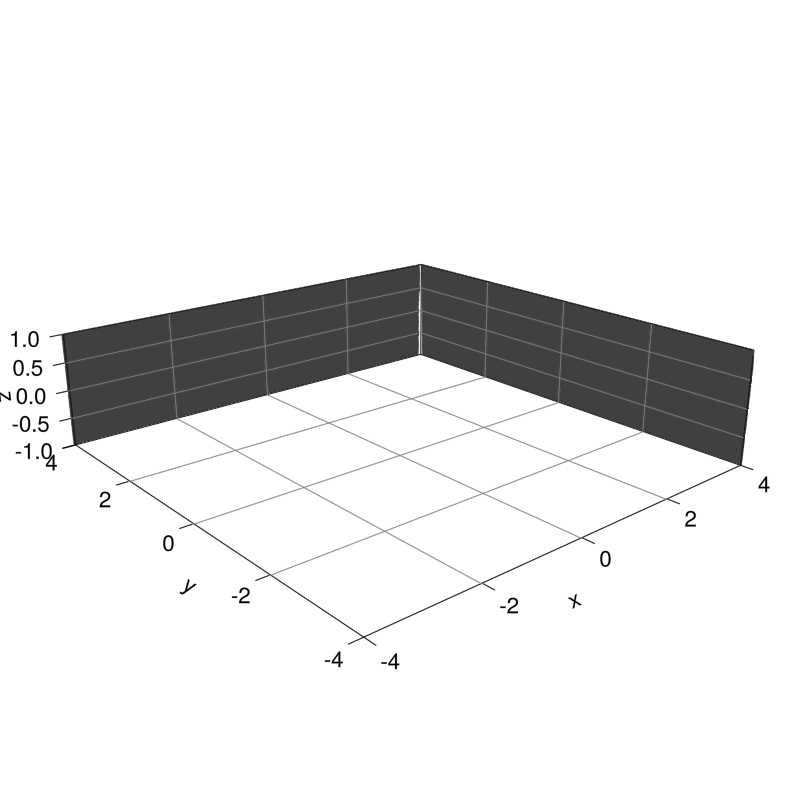# How to Call Function to Create Animation in GLMakie

Hi all,

I have a question, I don’t know how to call function so it can create the animation, I get the code here:

this is the code:

``````using GLMakie

Base.@kwdef mutable struct Torus
R::Float64 = 2
r::Float64 = 1
end

function generate_torus(torus::Torus, resolution=100; upto=1.0)
u = range(0, stop=2π*upto, length=resolution)
v = range(0, stop=2π*upto, length=resolution)
x = [ ( torus.R + torus.r*cos(vᵢ) ) * cos(uᵢ) for vᵢ in v, uᵢ in u ]
y = [ ( torus.R + torus.r*cos(vᵢ) ) * sin(uᵢ) for vᵢ in v, uᵢ in u ]
z = [ torus.r * sin(vᵢ) for vᵢ in v, _ in u ]
return x, y, z
end

function animate_torus(torus::Torus, filename="torus.gif")
fig = Figure(resolution = (500, 500))
ax = fig[1, 1] = LScene(fig)
cam3d!(ax.scene)

# Initialize surface plot with full torus
x, y, z = generate_torus(torus)
p = surface!(ax, x, y, z, colormap=:viridis, shading = false)
# Set the limits
xlims!(ax.scene, (-4, 4))
ylims!(ax.scene, (-4, 4))
zlims!(ax.scene, (-1, 1))

record(fig, filename, range(0, stop=1, length=100)) do i
# Update data of surface plot
x, y, z = generate_torus(torus, upto=i)
p = x
p = y
p = z
end
end
``````

the problem is when I type: `animate_torus(torus)` it returns UndefVarError: `torus` not defined and I think the poster in SoF will not answer it as quick as in Discourse, that is why I ask it here.

If your plot command (surface!) is outside the loop then you have to “update” it via observable.
See for example the paragraph [Animations using `Observables`] (Animations · Makie)

Here is the torus animation with observable. Note that you can have live plots and interactive plots in addition to the animation file production for free``````using GLMakie

Base.@kwdef mutable struct Torus
R::Float64 = 2
r::Float64 = 1
end

function generate_torus(torus::Torus,upto,resolution=100)
u = range(0, stop=2π*upto, length=resolution)
v = range(0, stop=2π*upto, length=resolution)
x = [ ( torus.R + torus.r*cos(vᵢ) ) * cos(uᵢ) for vᵢ in v, uᵢ in u ]
y = [ ( torus.R + torus.r*cos(vᵢ) ) * sin(uᵢ) for vᵢ in v, uᵢ in u ]
z = [ torus.r * sin(vᵢ) for vᵢ in v, _ in u ]
return (x, y, z)
end

function setup_scene(fig,torus::Torus,upto)
ax = Axis3(fig[1, 1], aspect = :data, perspectiveness = 0.5, elevation = π / 9,
xzpanelcolor = (:black, 0.75), yzpanelcolor = (:black, 0.75),
zgridcolor = :grey, ygridcolor = :grey, xgridcolor = :grey)
xlims!(ax, -4, 4)
ylims!(ax, -4, 4)
zlims!(ax, -1, 1)
lift(d->surface!( generate_torus(torus,d)...;  colormap=:plasma, shading = false,transparency = false),upto)
# lift(d->wireframe!(ax, generate_torus(torus,d)...; linewidth = 0.5, transparency = true),upto)
display(fig)
fig
end

function main_movie()
GLMakie.activate!()
fig = Figure(resolution = (800, 800), fontsize = 22)
upto = Observable(0.)
fig=setup_scene(fig,Torus(),upto)
# record(fig, "torus.mp4", range(0, stop=1, length=100)) do i
record(fig, "torus.gif", range(0, stop=1, length=100)) do i
upto[] = i # Update data of surface plot
end
end

function main_live()
GLMakie.activate!()
fig = Figure(resolution = (800, 800), fontsize = 22)
upto = Observable(0.)
fig=setup_scene(fig,Torus(),upto)
for i ∈  range(0, stop=1, length=100)
sleep(0.01)
upto[] = i # Update data of surface plot
end
end

function main_slider()
GLMakie.activate!(float=true)
fig = Figure(resolution = (2000, 2000), fontsize = 22)
sl = Slider(fig[2,1],range=0:0.01:1,startvalue=0.01)
fig = setup_scene(fig,Torus(),sl.value)
fig
end

main_live()
``````
2 Likes

So if there is code like this:

``````p = surface!(ax, x, y, z, colormap=:viridis, shading = false)
``````

I should add something like this then:

``````upto = Observable(0.)
``````

?

GLMakie is quite hard to grasp for beginner, but thanks for this, it helps a lot! Not only me but other people in this Discourse too.

Hi,

First I have to say that I am not the best specialist for Makie observable system, just a happy user, and it would be nice if a Makie specialist could review the proposed script (In particular, the main_live).

FWIW, my understanding of the observable system is:

1 : You define one (or several) observable for a given (or several) quantity that will change during the animation. Here the quantity is your torus parameter `upto`:

`````` upto = Observable(0.) #0 is the default value of the observable upto (here of type Observable(Int)).
``````

2 : If `o` is an observable, then `lift(x->f(x),o)` will execute the `f(new_value)` each time `o` is updated by the command `o[]=new_value`.

In the torus animation case this gives:

``````   record(fig, "torus.gif", range(0, stop=1, length=100)) do i
upto[] = i # Update the value of the upto observable with value i
end
``````

which triggers a new execution of the `surface!` Makie command via:

``````    lift(x->surface!( generate_torus(torus,x)...  ),upto)
``````

where the function `f(x)` function is in this case the following closure(*) :
`surface!( generate_torus(torus,x)... )`

Hope it helps.

Not completely trivial to grasp but quite powerful (IMHO). You could for example add a title to the figure showing the current value of the parameter `upto`. To achieve this you need to add another `lift` construction like `title = lift(x->string(x),upto)`.

I tried to illustrate that a useful consequence of the Observable system is to allow for different applications of the same code. In the torus case you have 3 different applications:

1. The animation via the `record` function (`main_movie`)
2. The `live` plot via a loop (`main_live`).
3. An interactive plot using `slider` widgets (`main_interactive`).

(*) closure : function that captures some context variables like `torus` in this case.

1 Like